Updating search results...

# 12 Results

View
Selected filters:
• Triple Threat, Inc.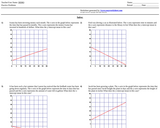Educational Use
Rating
0.0 stars

This resource provides word problems where students will need to explain linear models.

Subject:
Math 1
Mathematics
Material Type:
Interactive
Provider:
Triple Threat, Inc.
Author:
Easy Worksheet
04/04/1998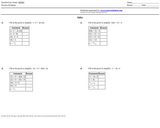Educational Use
Rating
0.0 stars

This resource provides problems for students to justify each solution method for linear equations with mathematical reasoning.

Subject:
Math 1
Mathematics
Material Type:
Interactive
Provider:
Triple Threat, Inc.
Author:
Easy Worksheet
04/04/1998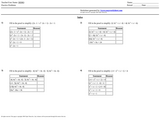Unrestricted Use
CC BY
Rating
0.0 stars

This resource provides problems to justify a solution method and to explain each step of the process using mathematical reasoning.

Subject:
Math 3
Mathematics
Material Type:
Lesson Plan
Provider:
Triple Threat, Inc.
Author:
Easy Worksheet
04/04/1998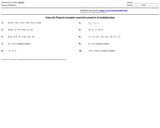Educational Use
Rating
0.0 stars

This resource contains exercises where students can be assessed on identifying properties in complex polynomial equations.

Subject:
Math 3
Mathematics
Material Type:
Lesson Plan
Provider:
Triple Threat, Inc.
Author:
Easy Worksheet
04/04/1998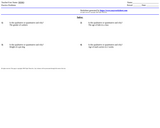Educational Use
Rating
0.0 stars

This resource is a worksheet that provides questions for students to solve to distinguish between quantitative and qualitative data.

Subject:
Math 3
Mathematics
Material Type:
Assessment
Provider:
Triple Threat, Inc.
Author:
EasyWorksheet
04/04/1998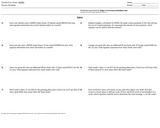Unrestricted Use
CC BY
Rating
0.0 stars

This resource provides word problems that will have students come up with equations with one variable to represent linear relationships to real world problems.

Subject:
Math 1
Mathematics
Material Type:
Activity/Lab
Provider:
Triple Threat, Inc.
Author:
Easy Worksheet
04/04/1998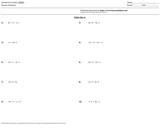Educational Use
Rating
0.0 stars

This resource provides problems for students to practice solving for one variable in a linear equation on both sides.

Subject:
Math 3
Mathematics
Material Type:
Lesson Plan
Provider:
Triple Threat, Inc.
Author:
Easy Worksheet
04/04/1998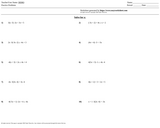Unrestricted Use
CC BY
Rating
0.0 stars

This resource provides problems where students can solve linear inequalities with one variable.

Subject:
Math 1
Mathematics
Material Type:
Activity/Lab
Provider:
Triple Threat, Inc.
Author:
Easy Worksheet
04/04/1998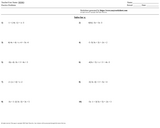Educational Use
Rating
0.0 stars

This resource provides exercises to solve multi-step linear equations in terms of x when it the constant (x) appears on both sides of the equation.

Subject:
Math 3
Mathematics
Material Type:
Lesson Plan
Provider:
Triple Threat, Inc.
Author:
Easy Worksheet
04/04/1998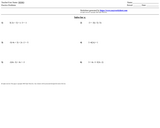Educational Use
Rating
0.0 stars

This resource provides problems for students to solve for multi-step linear equations.

Subject:
Math 3
Mathematics
Material Type:
Lesson Plan
Provider:
Triple Threat, Inc.
Author:
Easy Worksheet
04/04/1998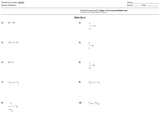Unrestricted Use
CC BY
Rating
0.0 stars

This resource provides problems for students to solve simple linear equations in terms of x.

Subject:
Math 3
Mathematics
Material Type:
Lesson Plan
Provider:
Triple Threat, Inc.
Author:
Easy Worksheet
04/04/1998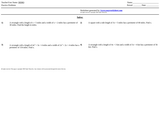Educational Use
Rating
0.0 stars

This resource provides problems where students can solve for x and verify that the set of values for width and length yield the specified perimeter of the rectangle. Students must be familiar with the definition of a perimeter.

Subject:
Math 1
Mathematics
Material Type:
Activity/Lab
Provider:
Triple Threat, Inc.
Author:
Easy Worksheet